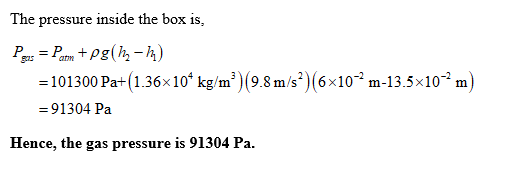# Assume patmos=1.00atm. what is the gas pressure pgas?

Assume patmos=1.00atm. What is the gas pressure pgas? Express
your answer in pascals to three significant figures. h1=13.5 cm h2=6.00 cm mercury of density= 1.36×104 kg/m3
4